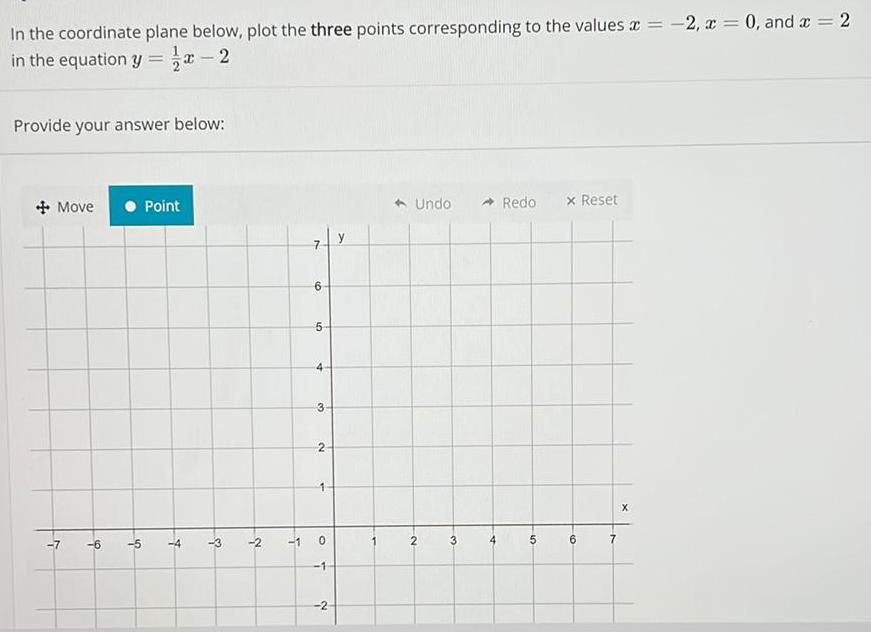Question:

# In the coordinate plane below, plot the three points

Last updated: 8/14/2022In the coordinate plane below, plot the three points corresponding to the values x = -2, x = 0, and x = 2 in the equation y = x-2.